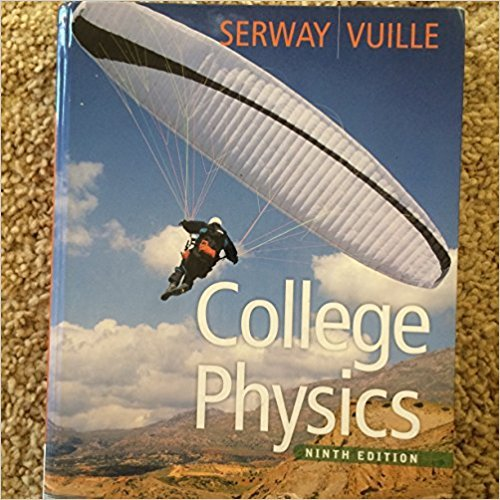×
Log in to StudySoup
Get Full Access to College Physics - 9 Edition - Chapter 23 - Problem 1
Join StudySoup for FREE
Get Full Access to College Physics - 9 Edition - Chapter 23 - Problem 1

Already have an account? Login here
×
Reset your password

# When an image of a real object is formed by a flat mirror,ISBN: 9780840062062 220

## Solution for problem 1 Chapter 23

College Physics | 9th Edition

• Textbook Solutions
• 2901 Step-by-step solutions solved by professors and subject experts
• Get 24/7 help from StudySoup virtual teaching assistantsCollege Physics | 9th Edition

4 5 1 265 Reviews
31
2
Problem 1

When an image of a real object is formed by a flat mirror, which of the following statements are always true? (a) The image is larger than the object. (b) The image is the same size as the object. (c) The image is virtual. (d) The image is smaller than the object. (e) The image is upright.

Step-by-Step Solution:
Step 1 of 3

PH (111) Fall 2017 Practice for Test (1), Thursday September 7 th 1. Which of the following is a scalar quantity Speed, Velocity, Displacement, Acceleration, or none of them. 2. A certain physical quantity R is calculated using the formula: R = 4a² (b – c) where (a) is a speed and (b) and (c) are distances. What is the SI unit for R 3. Express the following statement as an algebraic expression: “There are 264 gallons in a one cubic meter container.” Let G represent the number of gallons and M represent the number of one cubic meter containers. 4. Gold can be compressed to a thickness of 1.0 micron to make gold leaf. If each cubic centimeter of gold has a mass of 19.32 g, what

Step 2 of 3

Step 3 of 3

##### ISBN: 9780840062062

Unlock Textbook Solution

Enter your email below to unlock your verified solution to:

When an image of a real object is formed by a flat mirror,